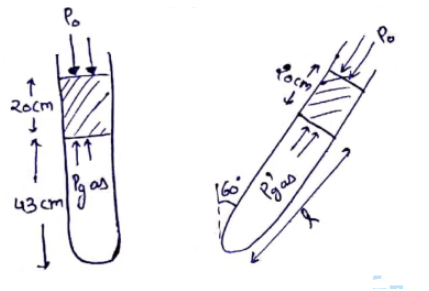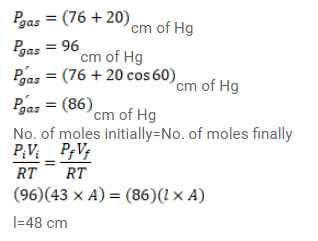# Use R=8.3 J/mol-K wherever required. An ideal gas is trapped between a mercury columnQuestion:

Use $\mathrm{R}=8.3 \mathrm{~J} / \mathrm{mol}-\mathrm{K}$ wherever required.

An ideal gas is trapped between a mercury column and the closed end of a narrow vertical tube of uniform base containing the column. The upper end of the tube is open to the atmosphere. The atmospheric pressure equals $76 \mathrm{~cm}$ of mercury. The lengths of the mercury column and the trapped air column are $20 \mathrm{~cm}$ and $43 \mathrm{~cm}$ respectively. What will be the length of the air column when the tube is titled slowly in a vertical plane through an angle of $60^{\circ} \mathrm{C}$ ? Assume the temperature to remain constant.

Solution: